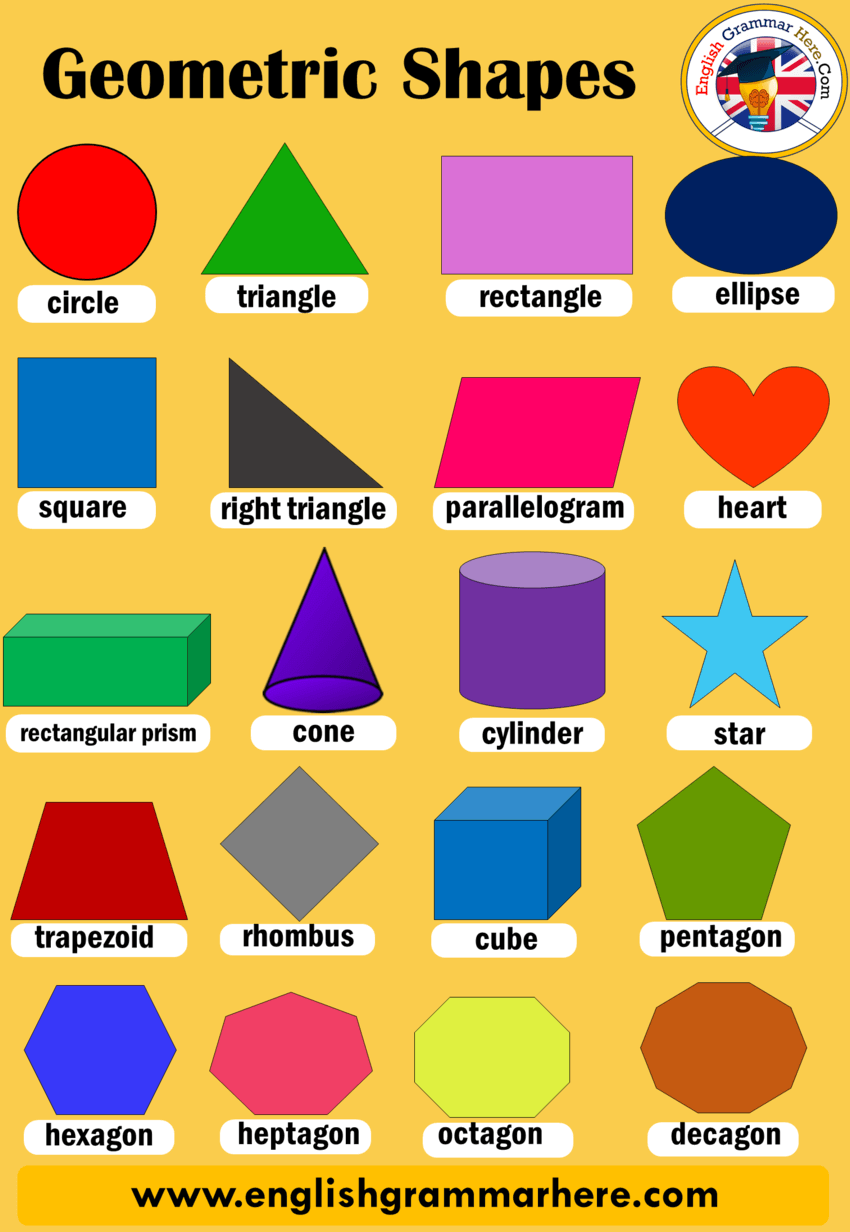# Geometric Shapes Names

English Geometric Shapes Names, Definition and Examples;##### Geometric Shapes List
• Nonagon
• Ellipse
• Rectangular
• Rectangular Prism
• Octagon
• Cone
• Triangle
• Scalene triangle
• Arrow
• Cube
• Cylinder
• Star
• Heptagon
• Hexagon
• Crescent
• Pyramid
• Right triangle
• Parallelogram
• Rhombus
• Square
• Pentagon
• Trapezoid
• Circle
• Oval
• Decagon
• Heart
• Cross

(For much more shapes names)

Geometric shapes can be named as figure or area closed by a boundary which is created by combining the specific amount of curves, points, and lines. There are different geometric shapes are Triangle, Circle, Square, etc. It is very important that you acquire the necessary understanding of the geometric shapes. For example; the common shapes in geometry like are square, rectangle, circle, and triangle. Here are some of geometric shapes and definition;

##### Square

A square is a four-sided area which is created by connecting 4 line segments. The line segments in the square are all of the equal lines. They come together to form 4 right angles.

##### Circle

If we talk about a circle which is another shape of geometry has no straight lines. It is rather a combination of curves that are all linked. In a circle, there is no angle to be found.

##### Rectangle

Similar to a square, a rectangle is also created by linking four line segments. However, there is only a difference between a square and a rectangle. In addition a rectangle, has two line segments which are longer than the other two line segments. So, in geometry, a rectangle is also called as an elongated square.

##### Triangle

Triangle comprises three linked line segments. Unlike, a square, in a triangle, the angles can be of distinct measurements. They aren’t always the right angles. Triangles are called, depending upon the type of angles which is found within the triangle itself. For example, if a triangle has one right angle, it will be known as a right-angled triangle.

However, in case all the angles of a triangle are less than 90 degrees, it will be named as an acute-angled triangle. If any, one of the angles in the triangle measures more than 90 degrees, then it will be known as an obtuse angled triangle. Finally, there is an equiangular triangle, in which all the angles of the triangle are 60 degrees. On the other hand, the triangle can also be identified or labeled on the type of sides they have. A scalene triangle has no congruent sides. An isosceles triangle has two congruent sides. An equilateral triangle has three congruent sides.